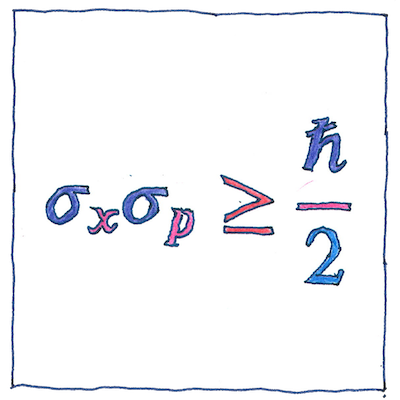# 1927

## The book of science

Tom Sharp

 Werner Heisenberg quantum mechanics

## Uncertainty principle

• Werner Heisenberg declared a limit
• to what can be determined precisely.
• We don’t have this problem
• when talking about planets or billiard balls,
• only for elementary particles
• such as photons or protons.
• The more we know about one property of a particle,
• such as its position,
• the less we know about any related property,
• such as its momentum.

## Half ℏ

• The standard deviations
• of position and momentum
• multiplied together
• is greater than or equal to
• half the reduced Planck constant.

## Unsure

• You’d think
• this’d be easy
• but I’m not sure.

Heisenberg’s uncertainty principle is a fundamental property of quantum systems, not merely a limit to how small a thing we can measure.

The illustration represents the work of Earle Hesse Kennard and Hermann Weyl. The standard deviation of position (sigma sub-x) multiplied by the standard deviation of momentum (sigma sub-p) is greater than or equal to half the reduced Planck constant.